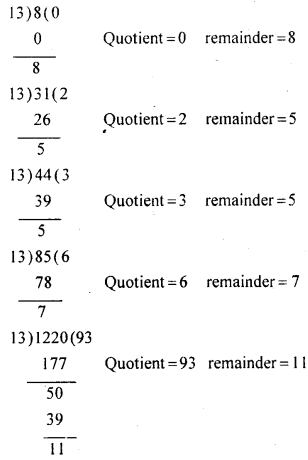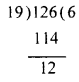# KSEEB Solutions for Class 8 Maths Chapter 1 Playing with Numbers Ex 1.3

Students can Download Maths Chapter 1 Playing with Numbers Ex 1.3 Questions and Answers, Notes Pdf, KSEEB Solutions for Class 8 Maths helps you to revise the complete Karnataka State Board Syllabus and score more marks in your examinations.

## Karnataka Board Class 8 Maths Chapter 1 Playing with Numbers Ex 1.3

Question 1.
Find the quotient and remainder when each of the following number is divided by 13 : 8, 31, 44, 85, 1220.Question 2.
Find the quotient and the remainder when each of the following numbers are divided by 304.
128, 636, 785, 1038, 2236, 8858Question 3.
Find the least natural number larger than 100 which leaves the remainder 12 when divided by 19.